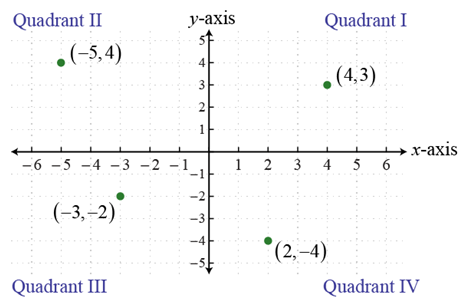# Section 2.1 - Rectangular Coordinates, Graphing, and Circles

Section Objectives

1. Graph two-variable equations in the rectangular coordinate system.
2. Find the distance between two points in the rectangular coordinate system.
3. Determine the midpoint of two points.
4. Use the standard form equation of a circle.
5. Graph circles.
6. Gain familiarity with some basic graphs.

### Rectangular Coordinate System

The rectangular (or Cartesian) coordinate system consists of two perpendicular number lines intersecting at their zero marks. The number lines are called axes---the horizontal axis is typically called the $x$-axis, while the vertical axis is the $y$-axis. The horizontal axis increases to the right; the vertical axis increases upward.

A point in the rectangular coordinate system is an ordered pair of coordinates, $(x,y)$, that indicates a location relative to the $x$- and $y$-axes. The point at the intersection of the number lines is called the origin and is given the coordinates $(0,0)$.A point is a solution of a two-variable equation if substitution of the coordinates for the corresponding variables yields a true statement.

The graph of a two-variable equation is the collection of all points that satisfy the equation.

For now, we will determine the graph of a two-variable equation by finding several solutions, plotting them, hoping they provide a representative sample, and generalizing to the overall graph from our sample.

#### Examples

• Find five (5) points that satisfy the equation $y=x-1$. Plot them and sketch the graph.

• Notice that the equation above is a linear equation (in two variables). Here is another: $x+2y=4$. Find some points that are solutions, and sketch the graph.

• Find five (5) points that satisfy the equation $y=x^2$. Plot them and sketch the graph.

• Based on the previous example, can you guess the graph of $y=x^2-1$.

• Find five (5) points that satisfy the equation $y=x^3$. Plot them and sketch the graph.

Important point: The graphs of linear equations, $y=x^2$, and $y=x^3$ are considered basic graphs. These are graphs you should quickly become familiar with.

### Midpoints and Distance

Think about two distinct points in the rectangular coordinate system. Imagine connecting them with a line segment. The midpoint of the segment is determined by finding the average of the coordinates:

The midpoint of the segment joining $(x_1,y_1)$ and $x_2,y_2)$ is given by $\displaystyle \left( \frac{x_1+x_2}{2}, \frac{y_1+y_2}{2} \right)$.

The length of the line segment or distance between the points can be obtained from the Pythagorean theorem:

Distance from $(x_1,y_1)$ to $(x_2,y_2) = \sqrt{(x_2-x_1)^2+(y_2-y_1)^2} = \sqrt{(\Delta x)^2+(\Delta y)^2}$.

### Examples

• Find the distance from $(0,0)$ to $(4,4)$. Write your answer in exact form.

• Determine the distance from $(-11,4)$ to $(-8,-6)$. Round to the nearest hundredth.

• In each example above, determine the midpoint of the given points.

### Circles

A circle is the set of all points in the plane (rectangular coordinate system) whose distance from a fixed point, called the center, is constant. The constant distance is called the radius of the circle.

It follows from the distance formula that the circle of radius $r$ centered at $(h,k)$ can be described by the standard form equation:

$(x-h)^2+(y-k)^2=r^2$.

#### Examples

• Find the standard form equation of the circle with center $(0,-1)$ and radius $4$.

• Graph the circle described by $(x-2)^2+(y+3)^2=12$.

• The equation $x^2+y^2+2x-4y-4=0$ describes a circle. Write the equation in standard form and determine the center and radius of the circle.